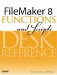# Cos()

Category: Trigonometric

 Syntax: Cos ( number )

Parameters:

numberAny expression that resolves to a numeric value that represents an angle measured in radians.

Data type returned: Number

Description:

Returns the cosine of the angle represented by the value of the parameter measured in radians. Cos is a periodic function with a range from -1 to 1.

In any right triangle, the cosine of the two non-right angles can be obtained by dividing the length of the side adjacent to the angle by the length of the hypotenuse.

You can convert an angle measured in degrees into radians by using the Radians() function, or by multiplying the value by Pi/180. One radian is slightly more than 57 degrees.

Examples:

``` Cos (0) = 0
Cos (Pi / 4) = .707106781 (which is 1 / Sqrt (2))
```FileMaker 8 Functions and Scripts Desk Reference
ISBN: 0789735113
EAN: 2147483647
Year: 2004
Pages: 352